# Classes Objects and Methods CS 5010 Program Design

• Slides: 18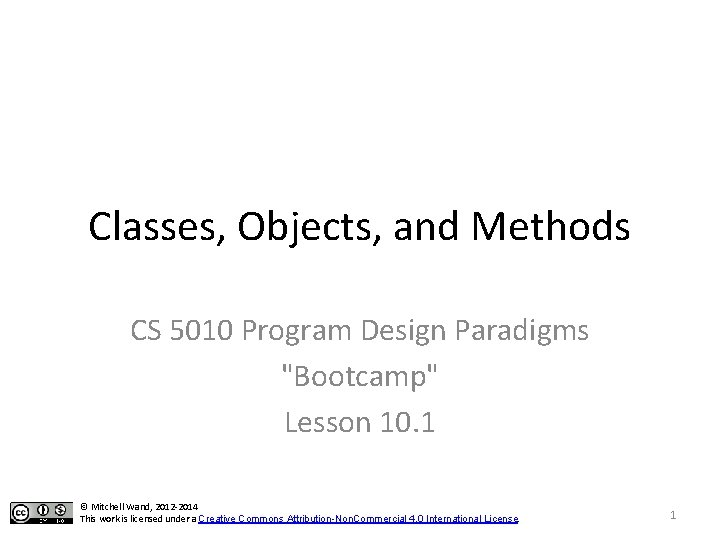Module Introduction • In this module, we will see how classes, objects, and interfaces fit into our account of information analysis and data design • We'll see how the functional and the objectoriented models are related • We'll learn how to apply the design recipe in an object-oriented setting. 2Goals of this lesson • Learn the basics about classes, objects, fields, and methods. • Learn how these ideas are expressed in the Racket object system 3What is an object? • An object is another way of representing compound data, like a struct. • Like a struct, it has fields. • It has one built-in field, called this, which always refers to this object • Here are pictures of two simple objects: x = 10 y = 20 r = 10 this = h = 30 w = 15 color = "blue" this = We assume that you've seen some kind of object-oriented programming before, so we're just reviewing vocabulary here. If you've really never used OOP before, go do some outside reading before continuing. 4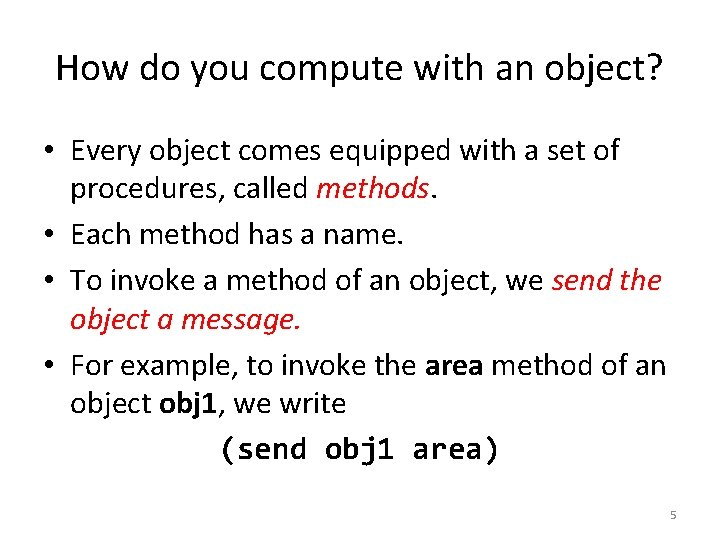How do you compute with an object? • Every object comes equipped with a set of procedures, called methods. • Each method has a name. • To invoke a method of an object, we send the object a message. • For example, to invoke the area method of an object obj 1, we write (send obj 1 area) 5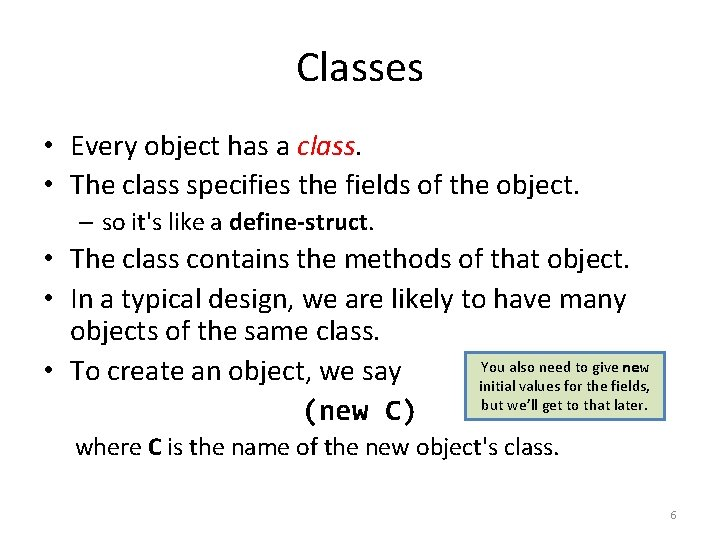Classes • Every object has a class. • The class specifies the fields of the object. – so it's like a define-struct. • The class contains the methods of that object. • In a typical design, we are likely to have many objects of the same class. You also need to give new • To create an object, we say initial values for the fields, but we’ll get to that later. (new C) where C is the name of the new object's class. 6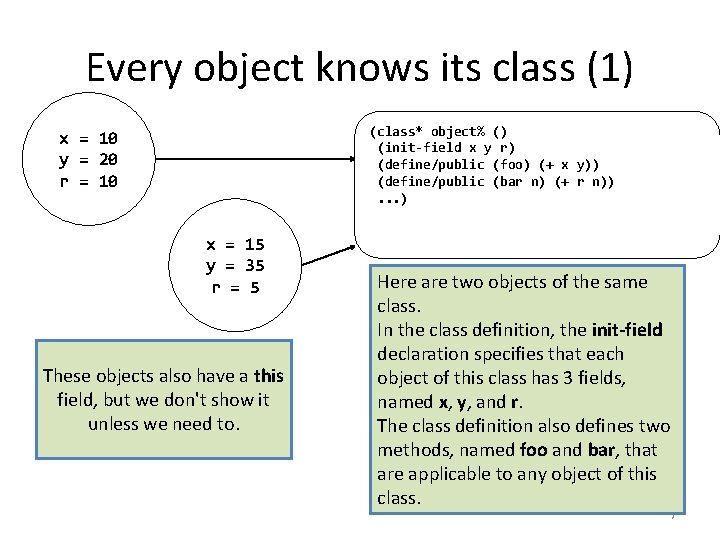Every object knows its class (1) (class* object% () (init-field x y r) (define/public (foo) (+ x y)) (define/public (bar n) (+ r n)). . . ) x = 10 y = 20 r = 10 x = 15 y = 35 r = 5 These objects also have a this field, but we don't show it unless we need to. Here are two objects of the same class. In the class definition, the init-field declaration specifies that each object of this class has 3 fields, named x, y, and r. The class definition also defines two methods, named foo and bar, that are applicable to any object of this class. 7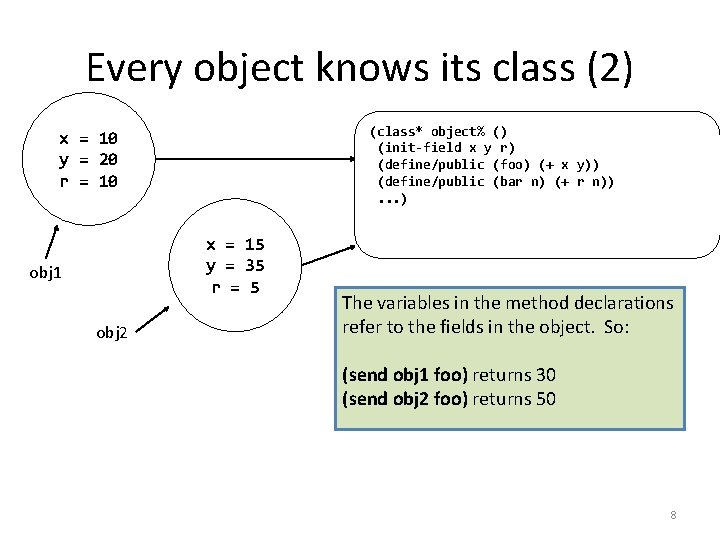Every object knows its class (2) (class* object% () (init-field x y r) (define/public (foo) (+ x y)) (define/public (bar n) (+ r n)). . . ) x = 10 y = 20 r = 10 x = 15 y = 35 r = 5 obj 1 obj 2 The variables in the method declarations refer to the fields in the object. So: (send obj 1 foo) returns 30 (send obj 2 foo) returns 50 8Every object knows its class (3) (class* object% () (init-field x y r) (define/public (foo) (+ x y)) (define/public (bar n) (+ r n)). . . ) x = 10 y = 20 r = 10 x = 15 y = 35 r = 5 obj 1 obj 2 Methods can also take arguments, just like functions. So (send obj 1 bar 8) returns 18 (send obj 2 bar 8) returns 13 9Every object knows its class (4) x = 10 y = 20 r = 10 this = x = 15 y = 35 r = 5 this = obj 1 obj 2 (class* object% () (init-field x y r) (define/public (foo) (+ x y)) (define/public (bar n) (+ r n)) (define/public (baz n) (+ (send this foo) n))). . . ) Methods are just Racket functions, so they can do anything a Racket function can do, including send messages to objects. (send obj 1 baz 20) returns (+ 30 20) = 50 (send obj 2 baz 20) returns (+ 50 20) = 70 10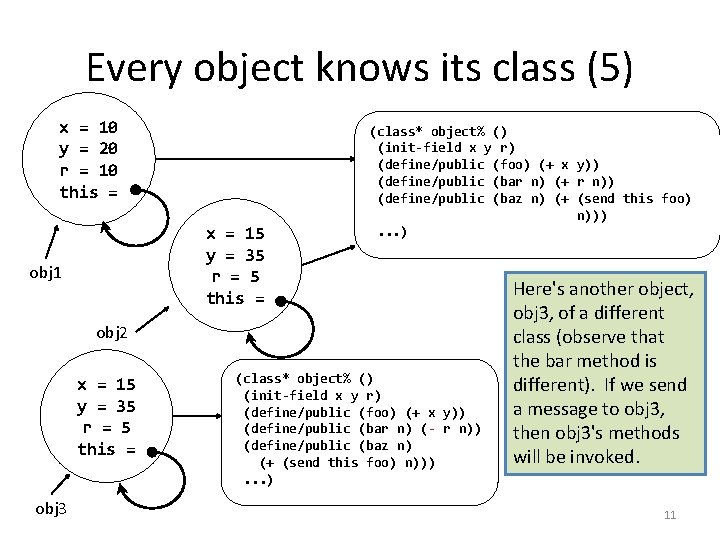Every object knows its class (5) x = 10 y = 20 r = 10 this = x = 15 y = 35 r = 5 this = obj 1 (class* object% () (init-field x y r) (define/public (foo) (+ x y)) (define/public (bar n) (+ r n)) (define/public (baz n) (+ (send this foo) n))). . . ) obj 2 x = 15 y = 35 r = 5 this = obj 3 (class* object% () (init-field x y r) (define/public (foo) (+ x y)) (define/public (bar n) (- r n)) (define/public (baz n) (+ (send this foo) n))). . . ) Here's another object, obj 3, of a different class (observe that the bar method is different). If we send a message to obj 3, then obj 3's methods will be invoked. 11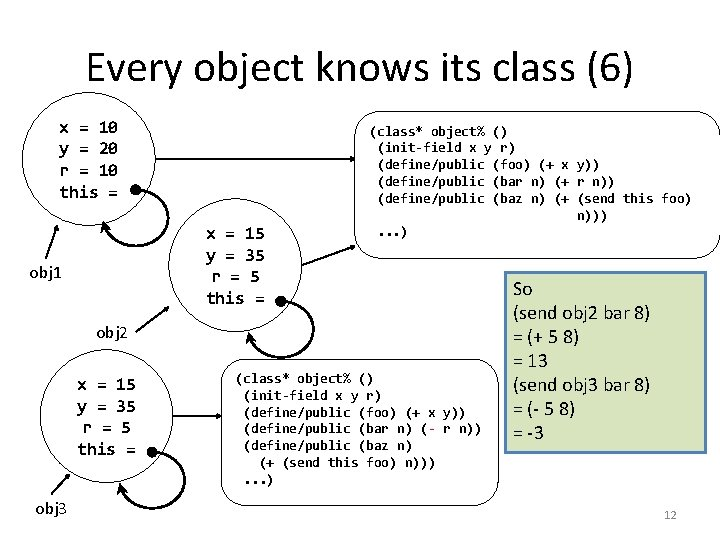Every object knows its class (6) x = 10 y = 20 r = 10 this = x = 15 y = 35 r = 5 this = obj 1 (class* object% () (init-field x y r) (define/public (foo) (+ x y)) (define/public (bar n) (+ r n)) (define/public (baz n) (+ (send this foo) n))). . . ) obj 2 x = 15 y = 35 r = 5 this = obj 3 (class* object% () (init-field x y r) (define/public (foo) (+ x y)) (define/public (bar n) (- r n)) (define/public (baz n) (+ (send this foo) n))). . . ) So (send obj 2 bar 8) = (+ 5 8) = 13 (send obj 3 bar 8) = (- 5 8) = -3 12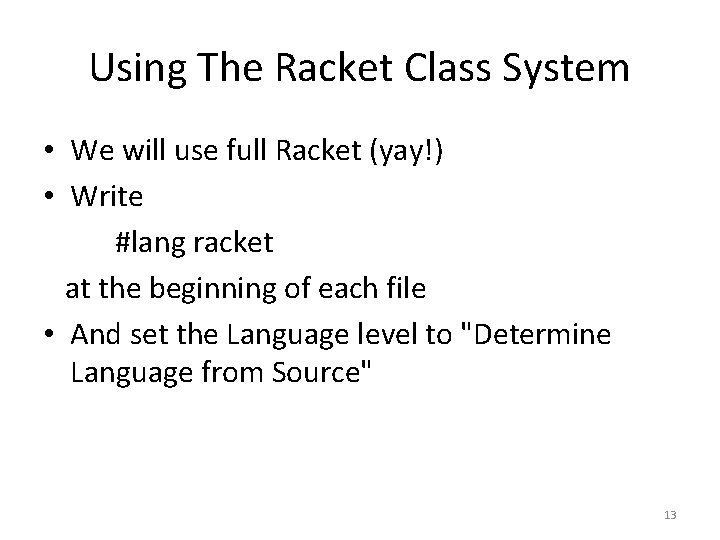Using The Racket Class System • We will use full Racket (yay!) • Write #lang racket at the beginning of each file • And set the Language level to "Determine Language from Source" 13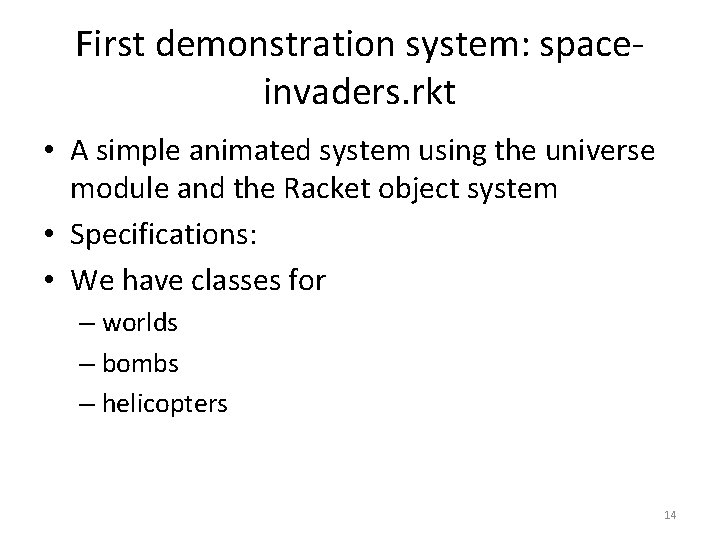First demonstration system: spaceinvaders. rkt • A simple animated system using the universe module and the Racket object system • Specifications: • We have classes for – worlds – bombs – helicopters 14Game Description • When the system starts, the world contains just a helicopter • the helicopter rises at a constant rate • Press space to drop a new bomb • Bombs fall at a constant rate • Bombs are draggable 15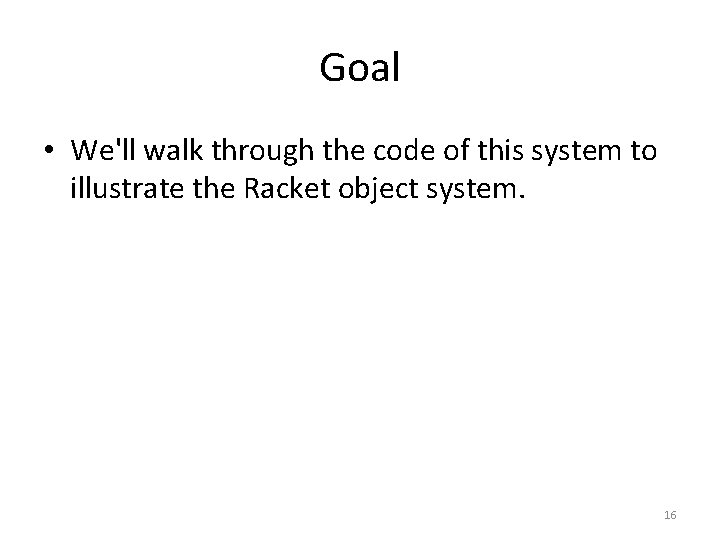Goal • We'll walk through the code of this system to illustrate the Racket object system. 16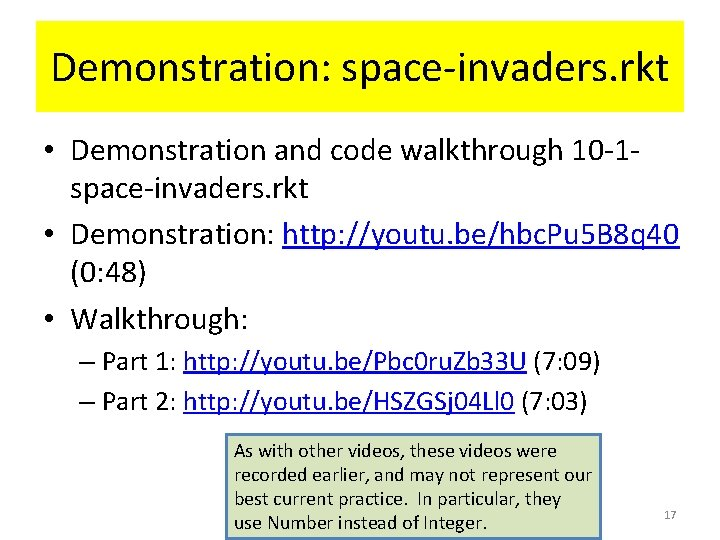Demonstration: space-invaders. rkt • Demonstration and code walkthrough 10 -1 space-invaders. rkt • Demonstration: http: //youtu. be/hbc. Pu 5 B 8 q 40 (0: 48) • Walkthrough: – Part 1: http: //youtu. be/Pbc 0 ru. Zb 33 U (7: 09) – Part 2: http: //youtu. be/HSZGSj 04 Ll 0 (7: 03) As with other videos, these videos were recorded earlier, and may not represent our best current practice. In particular, they use Number instead of Integer. 17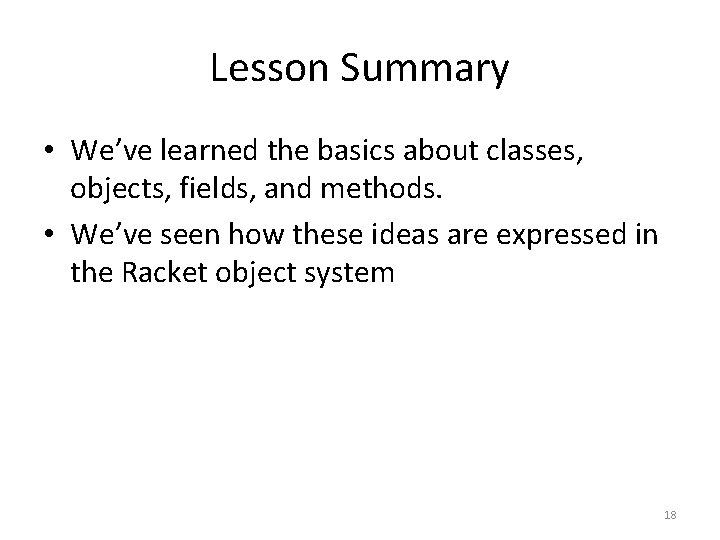Lesson Summary • We’ve learned the basics about classes, objects, fields, and methods. • We’ve seen how these ideas are expressed in the Racket object system 18